# Refraction of Light

12 de Mar de 2015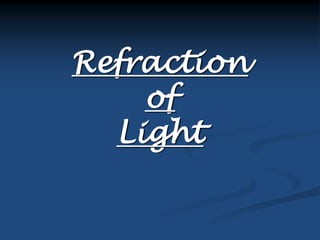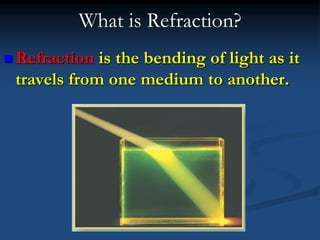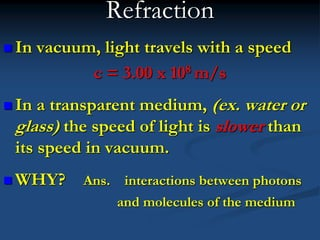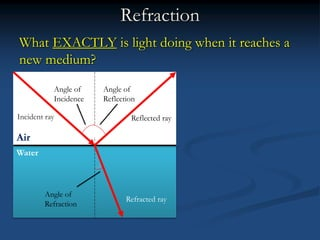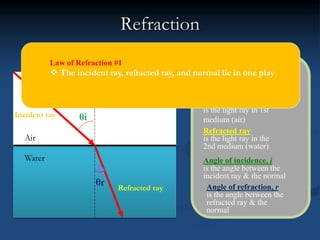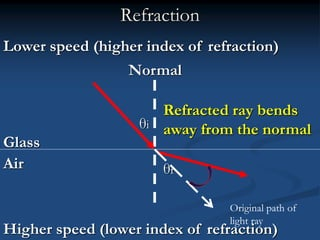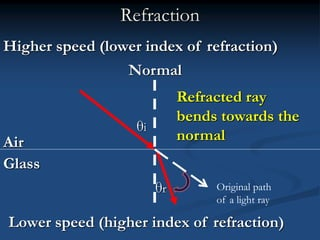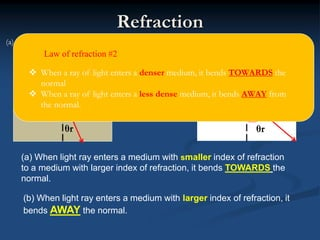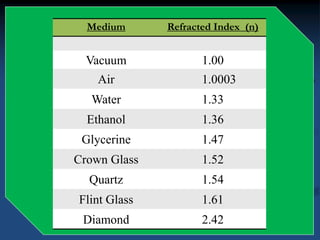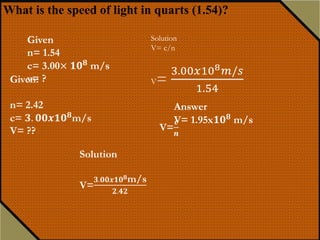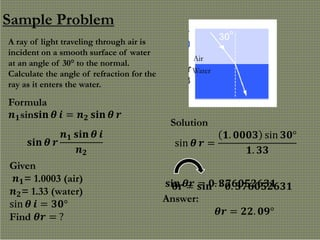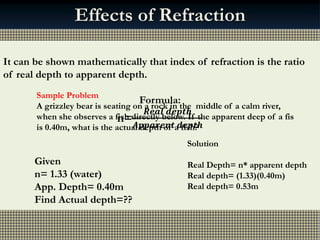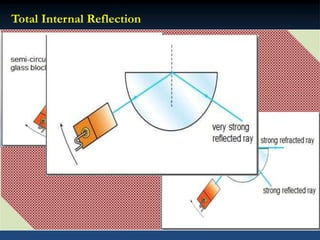1 de 14

### Refraction of Light

• 3. What is Refraction?  Refraction is the bending of light as it travels from one medium to another.
• 4. Refraction  In vacuum, light travels with a speed c = 3.00 x 108 m/s  In a transparent medium, (ex. water or glass) the speed of light is slower than its speed in vacuum.  WHY? Ans. interactions between photons and molecules of the medium
• 5. Refraction What EXACTLY is light doing when it reaches a new medium? Air Water Incident ray Reflected ray Refracted ray Angle of Incidence Angle of Reflection Angle of Refraction
• 6. Refraction Air Water Normal Incident ray Refracted ray θi θr Normal is a line drawn perpendicular to the surface Incident ray is the light ray in 1st medium (air) Refracted ray is the light ray in the 2nd medium (water) Angle of incidence, i is the angle between the incident ray & the normal Angle of refraction, r is the angle between the refracted ray & the normal Law of Refraction #1  The incident ray, refracted ray, and normal lie in one play.
• 7. Air Glass Normal Higher speed (lower index of refraction) Lower speed (higher index of refraction) Refraction θi θr Refracted ray bends away from the normal Original path of light ray
• 8. Refraction Air Glass Higher speed (lower index of refraction) Lower speed (higher index of refraction) Refracted ray bends towards the normal θi Normal θr Original path of a light ray
• 9. Refraction Air (a) Less dense to denser medium (b) Denser to less dense medium Air Glass Glass θi θr θi θr (a) When light ray enters a medium with smaller index of refraction to a medium with larger index of refraction, it bends TOWARDS the normal. (b) When light ray enters a medium with larger index of refraction, it bends AWAY the normal. Law of refraction #2  When a ray of light enters a denser medium, it bends TOWARDS the normal  When a ray of light enters a less dense medium, it bends AWAY from the normal.
• 10. What is “index of refraction”? The index of refraction (n) for a material is the ratio of the speed of light (c) in vacuum to that of the speed (v) in the medium. The ratio of the two speeds can be compared c n v  c v c n v  Medium Refracted Index (n) Vacuum 1.00 Air 1.0003 Water 1.33 Ethanol 1.36 Glycerine 1.47 Crown Glass 1.52 Quartz 1.54 Flint Glass 1.61 Diamond 2.42
• 11. Sample Problem What is the speed of light in a diamond if its index of refraction is 2.42? Light travels from air (n = 1) into glass, where its velocity reduces to only 2 x 108 m/s. What is the index of refraction for glass? vair = c vG = 2 x 108 m/s Glass Air 8 8 3 x 10 m/s 2 x 10 m/s c n v   Solution: Answer: n= 1.50 What is the speed of light in quarts (1.54)? Solution V= c/n V= 3.00𝑥108 𝑚/𝑠 1.54 Given n= 1.54 c= 3.00× 𝟏𝟎 𝟖 m/s v= ? Answer V= 1.95x𝟏𝟎 𝟖 m/s
• 12. Law of refraction 1. The incident ray, refracted ray, and normal lie in one plane. 2. When a ray of light enters a denser medium, it bends TOWARDS the normal. When a ray of light enters a less dense medium, it bends AWAY from the normal. 3. Snell’s Law is the relationship between the angle of incidence and angle of refraction and the angle of refraction of both materials. How can we figure out the angle of our refracted ray? We use Snell’s Law Willebrord van Roijen Snell (1580-1626) Sample Problem A ray of light traveling through air is incident on a smooth surface of water at an angle of 30° to the normal. Calculate the angle of refraction for the ray as it enters the water. Air Water 𝒏 𝟏sin𝐬𝐢𝐧 𝜽 𝒊 = 𝒏 𝟐 𝐬𝐢𝐧 𝜽 𝒓 Formula 𝐬𝐢𝐧 𝜽 𝒓 𝒏 𝟏 𝐬𝐢𝐧 𝜽 𝒊 𝒏 𝟐 Given 𝒏 𝟏= 1.0003 (air) 𝒏 𝟐= 1.33 (water) sin 𝜽 𝒊 = 𝟑𝟎° Find 𝜽𝒓 = ? Solution sin 𝜽 𝒓 = 𝟏. 𝟎𝟎𝟎𝟑 sin 𝟑𝟎° 𝟏. 𝟑𝟑 𝐬𝐢𝐧 𝜽𝒓 = 𝟎. 𝟑𝟕𝟔𝟎𝟓𝟐𝟔𝟑𝟏𝛉𝐫 = 𝐬𝐢𝐧−𝟏 𝟎. 𝟑𝟕𝟔𝟎𝟓𝟐𝟔𝟑𝟏 Answer: 𝜽𝒓 = 𝟐𝟐. 𝟎𝟗°
• 13. Mmmm..……. Fish and Chips!!! Normal Effects of Refraction Real depth Apparent depth To an observer standing at the side of a swimming pool, objects under the water appear to be nearer the surface than they really are. It can be shown mathematically that index of refraction is the ratio of real depth to apparent depth. Formula: n= 𝑹𝒆𝒂𝒍 𝒅𝒆𝒑𝒕𝒉 𝑨𝒑𝒑𝒂𝒓𝒆𝒏𝒕 𝒅𝒆𝒑𝒕𝒉 Sample Problem A grizzley bear is seating on a rock in the middle of a calm river, when she observes a fish directly below. If the apparent deep of a fis is 0.40m, what is the actual depth of a fish? Given n= 1.33 (water) App. Depth= 0.40m Find Actual depth=?? Solution Real Depth= n* apparent depth Real depth= (1.33)(0.40m) Real depth= 0.53m
• 14. Total Internal Reflection When light passes at an angle from a medium of higher index to one of lower index, the emerging ray bends away from the normal. Water Air light i = r Critical angle qc 900 When the angle reaches a certain maximum, it will be reflected internally. The critical angle is defined as the angle of incidence in the optically denser medium for which the angle of refraction in the optically less dense medium is 90°. n So the question is , how can you calculate the critical angle? Remember, it is when the refracted ray is equal to 90 degrees ɵc = sin-1 (𝒏 𝟐/𝒏 𝟏) Sample Problem What is the critical angle for water-air interface Given n1= (water) 1.33 n2= (air) 1.0003 𝜽𝒄 = sin−𝟏 𝟏. 𝟎𝟎𝟎𝟑 𝟏. 𝟑𝟑 𝜽𝒄 = 𝟒𝟖. 𝟕𝟕°

### Notas do Editor

1. Refraction is based on the idea that LIGHT is passing through one MEDIUM into another. It is the change in direction of a wave due to a change in its speed. It is most commonly observed when a wave passes from one medium to another. At the boundary between the media, the wave's phase velocity is altered, usually causing a change in direction The wavelength of the wave changes but its frequency remains constant.
2. What does this have to do with refraction? The difference in speed causes light rays to bend (refracted ) when traveling across different transparent materials!!!
3. So lets say for instance we have here a two medium (Click2) which is the air and water, and to see the boundary between the two medium we need to put a horizonal line and a vertical line to have or make a simple plane. Accordng to the law of reflecion Angle of incidence = Angle of Reflection Law of refraction Angle of Incidence > or < the Angle of refraction depending on the direction of the light Some of the light REFLECTS off the boundary and some of the light REFRACTS through the boundary
4. So we have here plane where in we have two meduim whch are the glass and air. The glass can lower the speed of light because It has a higher index of refracion compare with air that has a higher spedd of light but lower index of refraction.
5. Other problem!! Animaion An
6. A scientist by the name of Willebrord Snell discovered that the ratios of the index’s and the ratio of the sine of the angles are the same value! The fundamental law that governs the refraction of light is Snell's Law
7. Ok…… so how is knowing about refraction useful? An object seen in the water will usually appear to be at a different depth than it actually is, this is due to the refraction of light rays as they travel from the water into the air. The depth that the water appears to be when viewed from above is known as the apparent depth. While real depth it the actual depth. The apparent depth is less than the real depth because rays of light are refracted from the normal as they leave the water The eye, believing that light travels in straight lines, sees objects closer to the surface due to refraction. Such distortions are common.
8. There is a special type of refraction that can occur ONLY when traveling from a HIGH “n” medium to a LOW “n” medium. The angle of incidence is small therefore, it has a both reflected and refracted ray bThe angle of incidence is equal to the critical angle C. So the angle of refraction will be 90° and it also has a strong reflected ray The angle of incidence is larger than the critical angel. The angle of refraction will become smaller again. There will be no refracted ray. The light ray will be reflected the same way back. Summary: Total internal reflection takes place when light rays are travelling from an optically denser region to a less dense region, and the angle of incidence is greater than the critical angle.